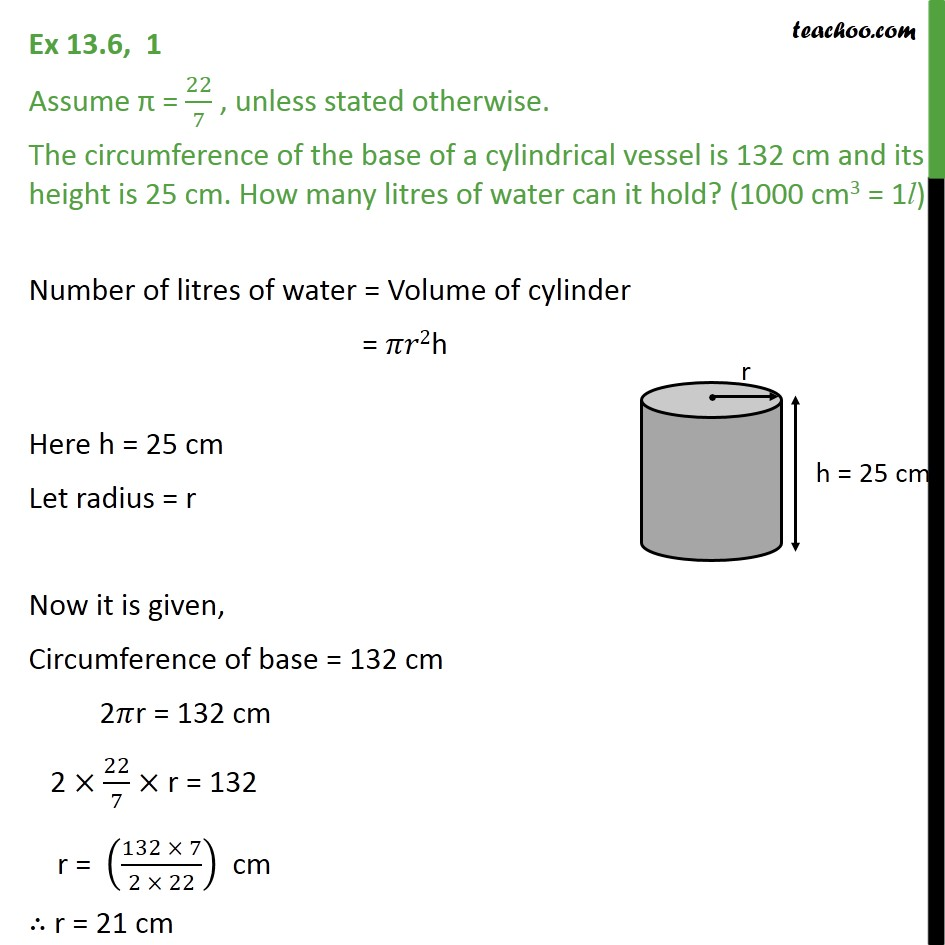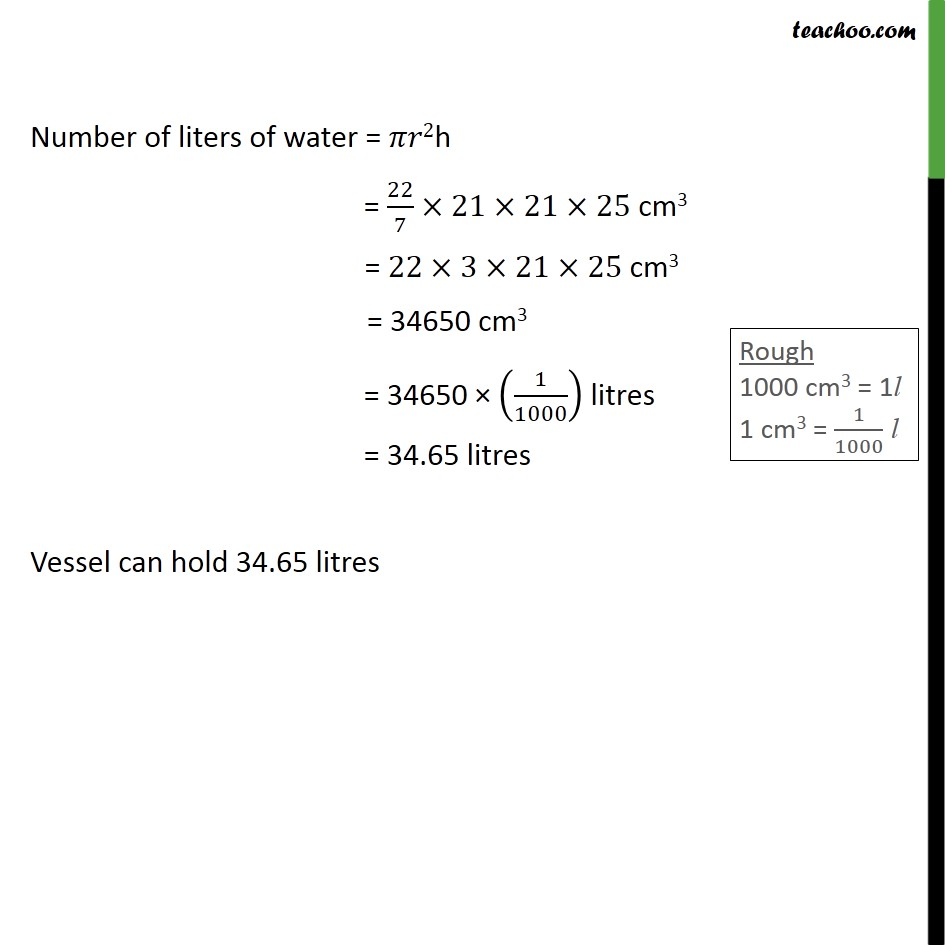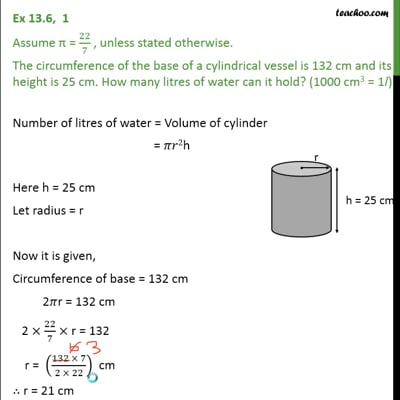Ex 13.6

Chapter 13 Class 9 Surface Areas and Volumes
Serial order wiseThis video is only available for Teachoo black users

Maths Crash Course - Live lectures + all videos + Real time Doubt solving!

### Transcript

Ex 13.6, 1 Assume = 22/7 , unless stated otherwise. The circumference of the base of a cylindrical vessel is 132 cm and its height is 25 cm. How many litres of water can it hold? (1000 cm3 = 1l) Number of litres of water = Volume of cylinder = 2h Here h = 25 cm Let radius = r Now it is given, Circumference of base = 132 cm 2 r = 132 cm 2 22/7 r = 132 r = ((132 7)/(2 22)) cm r = 21 cm Number of liters of water = 2h = 22/7 21 21 25 cm3 = 22 3 21 25 cm3 = 34650 cm3 = 34650 (1/1000) litres = 34.65 litres Vessel can hold 34.65 litres